MCAT Biology : Population Genetics and Hardy-Weinberg

Example Questions

Example Question #6 : Mendelian And Population Genetics

In a population of deer mice, the allele for white hair is recessive and the allele for brown hair is dominant. If the population consists of 500 individuals and the frequency of homozygous brown mice is 49%, what is the frequency of the recessive allele?

Assume the population is in Hardy-Weinberg equilibrium.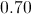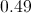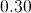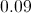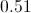Explanation:

In Hardy-Weinberg equilibrium, the sum of the dominant allele frequency (p) and the recessive allele frequency (q) is equal to 1.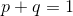The question says that 49% of the population consists of mice with the homozygous dominant gene, therefore, the dominant genotype frequency is equal to 0.49.

The question asks us to find the frequency of the recessive allele (q). In order to find the frequency of the recessive allele, we must first find the frequency of the dominant allele (p). According to the Hardy-Weinberg principle, the square root of the homozygous genotype frequency is equal to the allele frequency.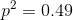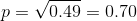The dominant allele frequency is 0.7. Using this, we can solve for the recessive allele frequency.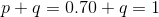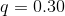Example Question #11 : Population Genetics And Hardy Weinberg

Which of the following is not a requirement for Hardy-Weinberg equilibrium?

Absence of migration

Random mating

Natural selection

Large population size

Natural selection

Explanation:

The following are requirements for Hardy-Weinberg.

• Organisms are diploid
• Only sexual reproduction occurs
• Generations are non overlapping
• Mating is random
• Population size is infinitely large
• Allele frequencies are equal in the sexes
• There is no migration, mutation, or selection

Natural selection would affect allele frequency in a population, thus disrupting Hardy-Weinberg equilibrium.

Example Question #11 : Population Genetics And Hardy Weinberg

Cystic fibrosis is an autosomal recessive disease. In a population of one hundred individuals, twenty-five are found to have the disease. Assuming Hardy-Weinberg equilibrium, what is the percent of the population that are carriers for cystic fibrosis?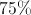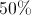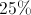Explanation:

The Hardy-Weinberg formulas tell us thatand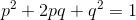, whereis the dominant allele frequency and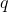is the recessive allele frequency.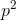is the frequency of homozygous dominant individuals in a population,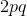is the frequency of heterozygous individuals, and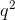is the frequency of homozygous recessive individuals.

In the question, we are told that 25 people out of 100 are homozygous recessive, meaning that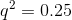.

Using this, we can find that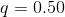.

If, and, then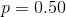.

We are looking for the frequency of heterozygous individuals ().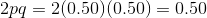50% of the population will be heterozygous (carriers) for the trait.

Example Question #13 : Population Genetics And Hardy Weinberg

In a population that is in Hardy-Weinberg equilibrium there is a gene that has only two alleles. If the dominant gene accounts for 70% of the gene pool, what percentage of the population is heterozygous for the trait?

49%

58%

42%

21%

42%

Explanation:

When a population is in Hardy-Weinberg equilibrium, we can predict the genotypic frequencies found in the population using the equation, whereis the frequency of the dominant allele, andis the frequency of the recessive allele. Sinceandaccount for the homozygotes in the population, we can find the frequency of the heterozygotes using theportion of the equation. We can first solve forusing the relationship.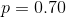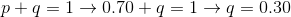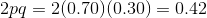Example Question #14 : Population Genetics And Hardy Weinberg

In a population that is in Hardy-Weinberg equilibrium, there is a gene that has only two alleles that codes for the color of the organism. Which of the following scenarios would not disrupt the Hardy-Weinberg equilibrium of the population?

A massive plague destroys 80% of the population

All color variations in the population have separate niches which allow them to thrive equally

One specific color is able to survive better in the population's present environment

Organisms begin to mate with partners that are the same color as themselves

All color variations in the population have separate niches which allow them to thrive equally

Explanation:

Hardy-Weinberg equilibrium is dependent on five key conditions:

1. The population is very large.

2. Mutations are in equilibrium (no net mutation rate).

3. There is no immigration/emigration that alters the gene pool ratios.

4. There is random mating.

5. There is no selection for the fittest organism taking place in the population.

If any of these conditions are violated in a population, Hardy-Weinberg equilibrium will be disrupted.

Example Question #15 : Population Genetics And Hardy Weinberg

75% of a given population test positive for Rhesus (Rh) factor antibodies. Given that blood type Rh+ is a dominant allele, what percentage of this population is homozygous?

We must know the total population size to solve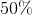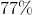Explanation:

This question is testing your knowledge of blood types and of the Hardy-Weinberg equations. Let's first evaluate the blood type portion of the question.

Rh-positive individuals will produce Rh factor. This molecule is an antigen. The given percentage refers to the number of individuals that produce antibodies to the Rh antigen. We can conclude that 75% of the population must be Rh-negative, since they produce antibodies against Rh factor; thus, 75% of the population must be homozygous recessive, since Rh-positive is a dominant allele.

Using this information, we can apply the Hardy-Weinberg equations:We know that 75% of the population is homozygous recessive, which corresponds to theterm in the second Hardy-Weinberg equation.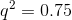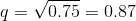Use this value to solve for the dominant allele frequency.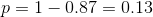The total percentage of the population that is homozygous will be given by the sum of theandterms in the Hardy-Weinberg equation.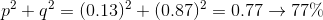Example Question #16 : Population Genetics And Hardy Weinberg

9% of a population of mice suffer from muscle spasms due to an autosomal recessive disease. What percentage of the population are carriers for the disease?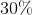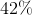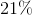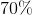Explanation:

This question requires application of the two Hardy-Weinberg equations:We know that the disease is autosomal recessive, which means that affected individuals must be homozygous. The frequency of the homozygous recessive genotype is given by theterm in the second Hardy-Weinberg equation. We can use the given genotype frequency to solve for the recessive allele frequency.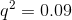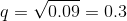Use the first Hardy-Weinberg equation to determine the dominant allele frequency.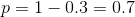The frequency of the heterozygous genotype (carriers) is given by theterm of the second Hardy-Weinberg equation. Use the values of the allele frequencies to calculate this term.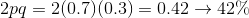Example Question #17 : Population Genetics And Hardy Weinberg

In a population of a particular island, 64% of individuals have a homozygous recessive genotype. Assuming the population correlates with Hardy-Weinberg principles, what percentage of individuals in the next generation will be heterozygous?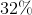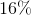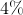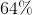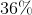Explanation:

Use equations p + q = 1 and p2 + 2pq + q2 = 1.

If 64% is homozygous recessive genotype, then  q= 0.64.

Then solve for p and q.

q = 0.8 and p = 0.2. The frequency of heterozygous individuals is 2pq or 2(0.2)(0.8) = 0.32

Example Question #11 : Population Genetics And Hardy Weinberg

A certain island-nation with a population of 200,000 has laws which severely restrict travel onto or off the island. There is an absolute prohibition against marrying foreigners, but island natives may marry as they wish. A non-lethal recessive genetic condition affects 2,000 of the people. How many carriers of this condition live on the island?

I.  198,000

II.  96,000

III.  36,000

IV.  12,400

V.  9,600

V

III

I

IV

II

III

Explanation:

The question assumes unusual conditions that must be met for a Hardy-Weinberg genetic equilibrium to exist. The population is large and isolated. The gene is assumed to be stable.  Mating is "random," but only within the population. Here, the affected homozygous recessive people number 2,000—one one hundredth of the population.

In Hardy-Weinberg terms, this means q squared is 0.01 and q (the frequency of the recessive allele) is 0.1.  Since p + q = 1, then p, the frequency of the dominant allele, must be 0.9. The carriers are denoted by 2pq because p + q = 1, and therefore p2 + 2pq + q2 is also equal to one. Here, 2 (0.9)(0.1) = 0.18, meaning that 18% of the 200,000 population, or 36,000 persons, are heterozygous carriers. The strict conditions for Hardy-Weinberg equilibrium are almost never satisfied in human populations.

Example Question #19 : Population Genetics And Hardy Weinberg

A botanist is reviewing a set of sequenced genomes from the tobacco plants he uses in his lab.  He notices frequency of plants that are phenotypically recessive is 0.16.  What are the frequencies of heterozygotes and homozygous dominant plants?

Heterozygotes = 0.40

Homozygous dominant = 0.60

Heterozygotes = 0.48

Homozygous dominant = 0.36

Heterozygotes = 0.60

Homozygous dominant = 0.40

Heterozygotes = 0.68

Homozygous dominant = 0.16

Heterozygotes = 0.36

Homozygous dominant = 0.48

We use the formula: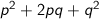where p and q are the allelic frequencies.  We know that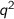is the frequency of homozygous recessive individuals, so q must be 0.4  That means that p must be 0.6, because p and q must add up to 1.  From there, we can just plug in 0.4 a 0.6 to get our answer.